﻿ 基于双剪统一屈服准则在岩质边坡稳定分析中的应用

# 基于双剪统一屈服准则在岩质边坡稳定分析中的应用Application of Double Shear Unified Yield Criterion to Stability Analysis of Rock Slope

Abstract: The Drucker-Prager yield criterion commonly used in rock slope analysis does not consider the intermediate principal stress effect and cannot reflect the real stress state of rock material. The double-shear unified strength theory contains a linear failure criterion which reflects the influence degree of intermediate principal stress by changing the intermediate principal stress coefficient. Using ANSYS finite element software based on double shear unified yield criterion and unified elastic-plastic finite element program UEPP, the rock slope stability was calculated as 0, 0.5 and 1 respectively. The results show that with the decrease of intermediate principal stress coefficient, the distribution area of equivalent plastic strain increases, the shear stress increases, and the bearing capacity of rock mass does not get greater play, which is not conducive to the practical engineering optimization design. In addition, in order to understand the influence of intermediate principal stress on slope stability analysis more clearly, the simulation results are compared with Drucker-Prager yield criterion. The results show that the intermediate principal stress cannot be ignored in slope stability analysis, and the rock slope based on the unified yield criterion of double shear is more difficult to penetrate, which can make full use of the strength potential of materials.

1. 引言

2. 基于统一强度理论的二次开发

2.1. 相关流动的双剪统一弹塑性刚度矩阵[Dep]

1985年，俞茂宏教授提出了新的双剪单元模型，由此给出了双剪理论 。在1990年俞茂宏教授增加了新的材料参数反映中间主应力效应，提出了统一强度理论，建立了一个新的双剪单元，认为作用于双剪单元上的两个较大剪应力及其面上的正应力所组成的函数到达一定的极限值时，材料发生破坏，其数学表达式为：

$\begin{array}{l}F={\tau }_{13}+b{\tau }_{12}+\beta \left({\sigma }_{13}+b{\sigma }_{12}\right)=c\\ {\tau }_{12}+\beta {\sigma }_{12}\ge {\tau }_{23}+\beta {\sigma }_{23}\\ {F}^{\prime }={\tau }_{13}+b{\tau }_{23}+\beta \left({\sigma }_{13}+b{\sigma }_{23}\right)=c\\ {\tau }_{12}+\beta {\sigma }_{12}\le {\tau }_{23}+\beta {\sigma }_{23}\end{array}$ (1)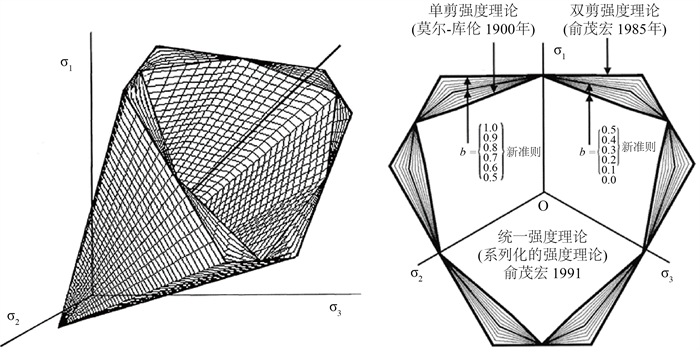Figure 1. Limit lines of unified strength theory on $\pi$ plane

$\begin{array}{ll}F=\left(1-\alpha \right)\frac{{I}_{1}}{3}+\frac{\alpha \left(1-b\right)}{1+b}\sqrt{{J}_{2}}\mathrm{sin}\theta +\left(2+\alpha \right)\sqrt{\frac{{J}_{2}}{3}}\mathrm{cos}\theta ={\sigma }_{t},\hfill & \left(0˚\le \theta \le {\theta }_{b}\right)\hfill \\ {F}^{\prime }=\left(1-a\right)\frac{{I}_{1}}{3}+\left(a+\frac{b}{1+b}\right)\sqrt{{J}_{2}}\mathrm{sin}\theta +\left(\frac{2-b}{1+b}+\alpha \right)\sqrt{\frac{{J}_{2}}{3}}\mathrm{cos}\theta ={\sigma }_{t},\hfill & \left({\theta }_{b}\le \theta \le 60˚\right)\hfill \end{array}$ (2)

${C}_{1}=\frac{\partial F}{\partial {I}_{1}}$ (3)

${C}_{2}=\frac{\partial F}{\partial \sqrt{{J}_{2}}}+\frac{c\mathrm{tan}3\theta }{\sqrt{{J}_{2}}}\frac{\partial F}{\partial \theta }$ (4)

${C}_{3}=-\frac{\sqrt{3}}{2\sqrt{{J}_{2}^{3}}\mathrm{sin}3\theta }\frac{\partial F}{\partial \theta }$ (5)

$F\ge {F}^{\prime }$ ，即 $0\le \theta \le {\theta }_{0}$

$\begin{array}{l}\underset{_}{{C}_{1}}=\frac{1}{3}\left(1-\alpha \right)\\ {C}_{2}=\left(1+\frac{\alpha }{2}\right)\frac{2}{\sqrt{3}}\mathrm{cos}\theta +\frac{\alpha \left(1-b\right)}{1+b}\mathrm{sin}\theta +\mathrm{cot}3\theta \left[-\left(1+\frac{\alpha }{2}\right)\frac{2}{\sqrt{3}}\mathrm{sin}\theta +\frac{\alpha \left(1-b\right)}{1+b}\mathrm{cos}\theta \right]\\ {C}_{3}=-\frac{\sqrt{3}}{2{J}_{2}\mathrm{sin}3\theta }\left[-\left(1+\frac{\alpha }{2}\right)\frac{2}{\sqrt{3}}\mathrm{sin}\theta +\frac{\alpha \left(1-b\right)}{1+b}\mathrm{cos}\theta \right]\end{array}$ (6)

$F<{F}^{\prime }$${\theta }_{0}<\theta \le \frac{\pi }{3}$

$\begin{array}{l}{{C}^{\prime }}_{1}=\frac{1}{3}\left(1-\alpha \right)\\ {{C}^{\prime }}_{2}=\left(\frac{2-b}{1+b}+\alpha \right)\frac{1}{\sqrt{3}}\mathrm{cos}\theta +\left(\alpha +\frac{b}{1+b}\right)\mathrm{sin}\theta +\mathrm{cot}3\theta \left[-\left(\frac{2-b}{1+b}+\alpha \right)\frac{1}{\sqrt{3}}\mathrm{sin}\theta +\left(\alpha +\frac{b}{1+b}\mathrm{cos}\theta \right)\right]\\ {{C}^{\prime }}_{3}=-\frac{\sqrt{3}}{2{J}_{2}\mathrm{sin}3\theta }\left[-\left(\frac{2-b}{1+b}+\alpha \right)\frac{1}{\sqrt{3}}\mathrm{sin}\theta +\left(\alpha +\frac{b}{1+b}\right)\mathrm{cos}\theta \right]\end{array}$ (7)

2.2. 统一弹塑性有限元程序UEPP 

UEPP中采用的材料模型不只有岩土类材料，还可以适用于金属材料、塑料材料、混凝土材料等各种类型材料，因此，UEPP可以广泛的应用于化学工程、机械工程、铁道工程、航空工程、土木工程。UEPP程序包括UEPP-2D、UEPP-3D和UEPP-3DH。其中UEPP-2D程序可以对平面应力应变问题进行相应的弹塑性分析，它的荷载类型可分为集中荷载、体力和分布力三种荷载类型，采用波前法求解，同时可以求解10000个自由度以内的问题。本文将UEPP-2D程序添加到有限元软件ANSYS中进行后续的数值模拟，限于篇幅不再给出程序代码。图2为UEPP程序流程图。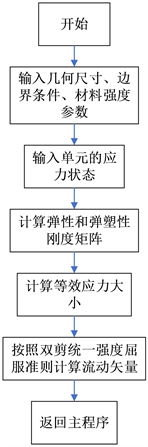Figure 2. Flowchart of UEPP program

3. 岩质边坡稳定性数值分析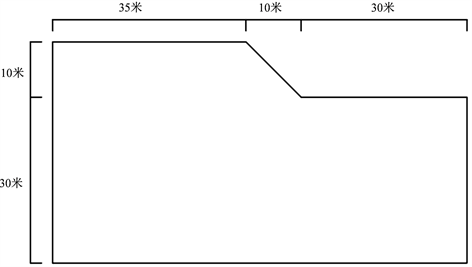Figure 3. Slope profile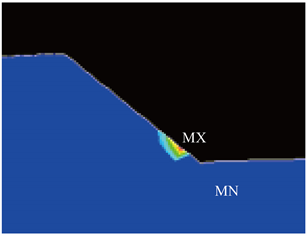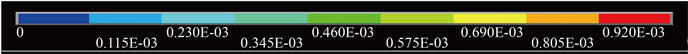(a) 等效塑性应变云图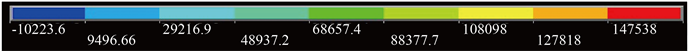(b) XY方向切应力

Figure 4. b = 0 the equivalent plastic strain cloud and XY direction shear stress cloud(a) 等效塑性应变图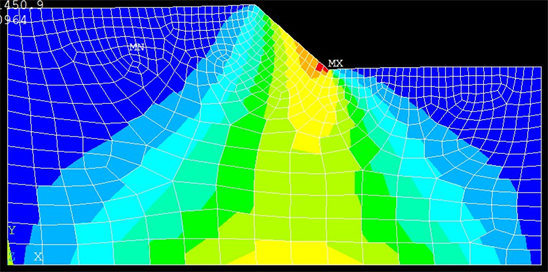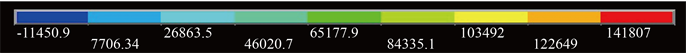(b) XY方向切应力

Figure 5. b = 0.5, the equivalent plastic strain cloud and XY direction shear stress cloud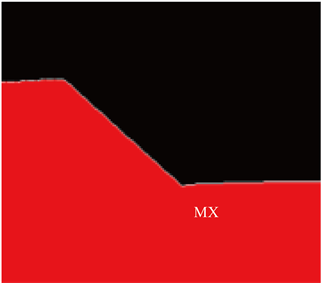(a) 等效塑性应变图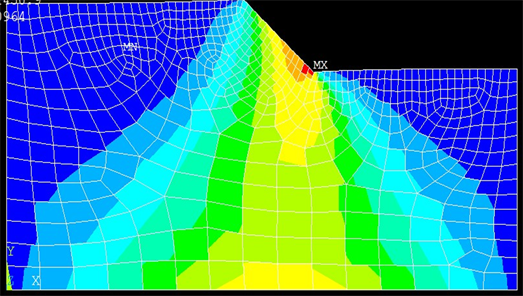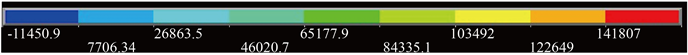(b) XY方向切应力

Figure 6. b = 1, the equivalent plastic strain cloud and XY direction shear stress cloud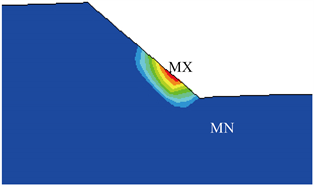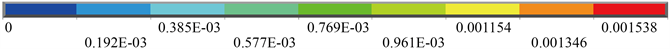Figure 7. Equivalent plastic strain diagram for Drucker-Prager materialTable 2. Comparison table of safety factor results

4. 结论

1) 随着中间主应力对边坡的破坏影响增大，边坡体的等效塑性应变与XY方向切应力变小，屈服强度增大，安全系数增大，边坡体不易贯通破坏。

2) 将不同主应力下的计算结果与Drucker-Prager准则的计算结果相比，安全系数偏小，D-P准则下的贯通性更明显，由此表明D-P强度准则下分析得到的边坡稳定性偏安全。可见D-P本构模型并没有充分发挥材料的潜力，在实际工程中会产生巨大的浪费。

NOTES

*通讯作者。

 王新敏. ANSYS工程结构数值分析[M]. 北京: 人民交通出版社, 2007.

 昝月稳. 岩石非线性统一强度理论及其在岩体工程中应用[D]: [博士学位论文]. 西安: 西安交通大学, 2001.

 宿辉, 党承华, 崔佳佳. 邓肯-张非线性模型研究及其在ANSYS中的实现[J]. 中国农村水利水电, 2010(3): 76-79.

 黄强, 黄宜胜, 肖志勇, 等. ANSYS中强度折减法等效DP-MC准则二次开发[J]. 人民黄河, 2016, 38(4): 94-96.

 袁野, 费文平. 基于ANSYS软件UPFs的邓肯-张模型二次开发[J]. 武汉大学学报(工学版), 2021, 54(7): 601-608.

 关云飞, 高峰, 赵维炳, 等. ANSYS软件中修正剑桥模型的二次开发[J]. 岩土力学, 2010, 31(3): 976-980.

 师访. ANSYS二次开发及应用实例详解[M]. 北京: 中国水利水电出版社, 2012.

 俞茂宏. 强度理论新体系: 理论、发展和应用[M]. 西安: 西安交通大学出版社, 2011.

 Yu, M.H., He, L.N. and Song, L.Y. (1985) Twin Shear Stress Theory and Its Generalization. Science in China, Series A, 11, 1174-1183.

 俞茂宏. 双剪理论及其应用[M]. 北京: 科学出版社, 1998.

 俞茂宏. 岩土类材料的统一强度理论及其应用[J]. 岩土工程学报, 1994(2): 1-10.

 俞茂宏, 昝月稳, 李建春. 统一强度理论角点奇异性的统一处理[J]. 岩石力学与工程学报, 2000(S1): 849-852.

Top